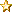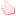###Author Topic: Variables Signed/Unsigned and such  (Read 138 times)

#### Nick_W

• Full Member
•• Posts: 215##### Variables Signed/Unsigned and such
« on: July 26, 2016, 05:30:09 pm »
Hi,

I'm converting a library from Arduino (C) for use with a sensor, and it's proving a right pain.

The main problem comes from trying to convert signed/unsigned bytes, shorts and ints using ZeroExtend() and SignExtend(). This makes things either an Integer or a Float, and is very difficult to deal with. Is there no easier way to represent these values? can we not introduce the concept of signed bytes and unsiged short/ints? it would make things so much simpler to convert from C.

Also my pressure transducer uses a 64 bit integer for full precision. I can use a  32 bit variable, if I reduce precision - but a coretx M0 or M3 can handle 64 bit integers, is there no way to represent an int64_t or uint64_t? Do I have to sacrifice precision because of this weird restriction?

The calculation (in C) is here:

Code: [Select]
`float readPressure(void) {  int64_t var1, var2, p;  readTemperature(); // must be done first to get t_fine  int32_t adc_P = read24(BME280_REGISTER_PRESSUREDATA);  adc_P >>= 4;  var1 = ((int64_t)t_fine) - 128000;  var2 = var1 * var1 * (int64_t)_bme280_calib.dig_P6;  var2 = var2 + ((var1*(int64_t)_bme280_calib.dig_P5)<<17);  var2 = var2 + (((int64_t)_bme280_calib.dig_P4)<<35);  var1 = ((var1 * var1 * (int64_t)_bme280_calib.dig_P3)>>8) +    ((var1 * (int64_t)_bme280_calib.dig_P2)<<12);  var1 = (((((int64_t)1)<<47)+var1))*((int64_t)_bme280_calib.dig_P1)>>33;  if (var1 == 0) {    return 0;  // avoid exception caused by division by zero  }  p = 1048576 - adc_P;  p = (((p<<31) - var2)*3125) / var1;  var1 = (((int64_t)_bme280_calib.dig_P9) * (p>>13) * (p>>13)) >> 25;  var2 = (((int64_t)_bme280_calib.dig_P8) * p) >> 19;  p = ((p + var1 + var2) >> 8) + (((int64_t)_bme280_calib.dig_P7)<<4);  return (float)p/256;}`

#### mc-John

• Global Moderator
• Full Member
•• Posts: 212##### Re: Variables Signed/Unsigned and such
« Reply #1 on: July 28, 2016, 10:02:03 am »
We currently don't support 64 bit integers but we will in the future. I downloaded the datasheet and there is a 64, 32 and double implementation of the functions. By the way this is a very nice sensor.

I have added the float implementation based on page 49 of the manual. Our floats are 6 digits accurate so that should be good enough for the accuracy of the sensor

Code: [Select]
`Class test12    Public Function BME280_compensate_P_double(adc_P As Integer) As Float        ' Variables below need to be set        Dim t_fine As Integer        Dim dig_P9 As Integer        Dim dig_P8 As Integer        Dim dig_P7 As Integer        Dim dig_P6 As Integer        Dim dig_P5 As Integer        Dim dig_P4 As Integer        Dim dig_P3 As Integer        Dim dig_P2 As Integer        Dim dig_P1 As Integer                Dim var1 As Float         Dim var2 As Float         Dim p As Float        var1 = t_fine / 2 - 64000.0        var2 = var1 * var1 * dig_P6 / 32768.0         var2 = var2 + var1 * dig_P5 * 2.0         var2 = (var2 / 4.0) + (dig_P4 * 65536.0)         var1 = (dig_P3 * var1 * var1 / 524288.0 + dig_P2 * var1) / 524288.0         var1 = (1.0 + var1 / 32768.0) * dig_P1        If (var1 = 0.0) Then            Return 0 // avoid exception caused by division by zero        End If        p = 1048576.0 - adc_P         p = (p - (var2 / 4096.0)) * 6250.0 / var1         var1 = dig_P9 * p * p / 2147483648.0         var2 = p * dig_P8 / 32768.0         p = p + (var1 + var2 + dig_P7) / 16.0         Return p     End Function    End Class `
Also there are some unsigned short in the BME280 registers. See 5.2.10 how to handle an unsigned short. In short the ZeroExtend method will create an Integer from an unsigned short to handle values greater than 32768.
« Last Edit: July 28, 2016, 10:26:04 am by mc-John »Useful x 1 View List

#### Nick_W

• Full Member
•• Posts: 215##### Re: Variables Signed/Unsigned and such
« Reply #2 on: July 28, 2016, 02:06:41 pm »
Thank you!I was aware of the 32 bit compensation function, and I implemented this. Nice to know 64 integers will be supported in the future.

I will compare your implementation with mine - I'm sure I will learn a lot.

I guess I'm just not used to dealing with all integers. I did the same thing as you and just converted all the calibration values to Integers.

This sensor is nice because the standby current (and active current for that matter) is so low, it makes a nice battery powered environmental sensor.

I'm reading temperature and humidity fine at the moment, but pressure is giving me weird results - probably due to the complicated maths, which I no doubt have wrong somewhere.

I'll let you know how it goes.

Thanks again.Like x 1 View List

#### Nick_W

• Full Member
•• Posts: 215##### Re: Variables Signed/Unsigned and such
« Reply #3 on: July 28, 2016, 05:27:43 pm »
OK,

After some testing, I have to say your pressure compensation routine does not work, it gives all sorts of random results. My routine also gives wrong results, but they are consistently wrong (ie it gives a large number which varies slightly with each reading). Yours gives medium positive or negative numbers which do not look like pressure readings, and vary a lot.

Still trying to track down the errors, the lack of a uint32_t makes life very hard! Not being able to do bit-wise arithmetic (because they get converted to Floats) is mind bendingly difficult. Some things just do not have a negative value...

This is my attempt:

Code: [Select]
`    Shared Function BME280_compensate_P_int32(adc_P As Integer) As Float        // Returns pressure in Pa as float. Output value of 96386 equals 96386 Pa = 963.86 hPa                Dim var1 As Integer        Dim var2 As Integer        Dim p As Integer        var1 = (t_fine >> 1) - 64000        var2 = (((var1 >> 2) * (var1 >> 2)) >> 11) * dig_P6        var2 = var2 + ((var1 * dig_P5) << 1)        var2 = (var2 >> 2) + (dig_P4 << 16)        var1 = (((dig_P3 * (((var1 >> 2) * (var1 >> 2)) >> 13)) >> 3) + ((dig_P2 * var1) >> 1)) >> 18        var1 = (((32768 + var1)) * dig_P1) >> 15        If var1 = 0 Then            Return 0 // avoid exception caused by division by zero        End If        p = ((1048576 - adc_P.ZeroExtend()) - (var2 >> 12)) * 3125        If p < 0x80000000 Then            p = (p << 1) / var1.ZeroExtend()        Else            p = (p / var1.ZeroExtend()) * 2        End If        var1 = (dig_P9 * ((((p >> 3) * (p >> 3)) >> 13))) >> 12        var2 = ((p >> 2) * dig_P8) >> 13        p = p + ((var1 + var2 + dig_P7) >> 4)        Return p.ZeroExtend()    End Function`
output is
Code: [Select]
`MCThings/70074/Pressure 1201306112.000000`
Which varies slightly, but is consistent.

I'll look at it again tomorrow, mind is too boggled to think straight now.

#### mc-Abe##### Re: Variables Signed/Unsigned and such
« Reply #4 on: July 29, 2016, 10:28:27 am »
Could you provide a set of sample values for adc_P and dig_P# variables? I would like to run a sample calculation and see if there is an issue in the code that runs behinds the scene or if there is a precision issue with only using a float and not a double.

#### Nick_W

• Full Member
•• Posts: 215##### Re: Variables Signed/Unsigned and such
« Reply #5 on: July 29, 2016, 04:25:05 pm »
I was going to do this, but I've been having all sorts of problems all day. I now seem to have bricked my module (can't connect, and just resets continually - really need the "erase flash RAM" option) .

Anyway, I've attached a zip with two projects in it (same project as I've been working on). Both have no compile errors, but one works, and one does not. The working one uses a shared object (so you don't have to instantiate it), the other (not working) uses public subs/functions/variables so you do.

Ignore all the calculations etc, What am I doing wrong on creating my object?

I have tried debugging to see if there is a run time error, but I can't get the "run" option to work (works on simple projects, just not complicated ones).

Any Help would be appreciated.

#### Nick_W

• Full Member
•• Posts: 215##### Re: Variables Signed/Unsigned and such
« Reply #6 on: August 18, 2016, 10:54:07 am »
Just FYI,

I now have this module working, with what look like sane values.

Your conversion function does work! it was my errors that were causing weird results. Many Thanks for the effort.

I now have a working class that supports the BME280 temp/humidity/pressure module.

I now need to work on the rest of the program....